# Efficient Wind Speed Forecasting Using Discrete Wavelet Transform and Artificial Neural Networks

Efficient Wind Speed Forecasting Using Discrete Wavelet Transform and Artificial Neural Networks
Fac. Sci. & Tec., LEER Lab., Univ. Souk Ahras, 41000, Algeria

Corresponding Author Email:
khaled.khelil@univ-soukahras.dz
Page:
447-452
|
DOI:
https://doi.org/10.18280/ria.330607
8 September 2019
|
Accepted:
2 November 2019
|
Published:
30 December 2019
| Citation

OPEN ACCESS

Abstract:

Electricity demand is globally increasing throughout the world, giving rise to many other renewable energy sources such as solar and wind energy. Therefore, to efficiently manage grid-connected wind power farms, precise wind forecast is very useful and necessary. This paper seeks to find out the most appropriate discrete wavelet transform (DWT), combined with artificial neural networks (ANN), for wind speed forecasting. Wavelet decomposition is applied to obtain a smoothed wind speed signal for more accurate prediction through neural networks. Wind speed data of three cities from the northern Africa region are employed, namely Annaba, Sidi Bouzid and Tetouan located in Algeria, Tunisia and Morocco respectively. The obtained simulation results show that the Daubechies wavelet db4 with 5-level decomposition outperforms all other standard wavelets in terms of wind speed forecasting accuracy.

Keywords:

wind power forecasting, discrete wavelet transform, neural networks

1. Introduction

Today renewable energies occupy a very important place in electrical energy production. Among them, wind energy which is growing considerably and continuously during recent years . having several advantages, such as mitigation of climate change, reduction of energy dependence, and contribution to sustainable progress of many developing countries, the installation estimation, throughout the world, of this kind of energy will achieve approximately 800 GW by the end of 2021 .

However, due to the irregular nature of wind energy production, its incorporation into the conventional electrical power system requires several technical and economic challenges [3-5]. To deal with the intermittency and randomness of wind energy conversion, forecasting techniques, over an extended period of time, are required . Various forecasting approaches, including physical, statistical, intelligent and hybrid methods have been proposed [1-3, 5, 6]. Although physical methods are effective in predicting the dynamics of the atmosphere, this method require a large amount of numerical weather forecast data such as temperature, humidity, speed, pressure, topological parameters, etc. This results in accumulation of data and this method need large computational time to correlate these data in order to forecast the wind speed. Due to this limitation, these methods are not suitable for short-term forecasting horizons; therefore， these approaches are best suited for medium-term and long-term wind speed forecasting [2, 7, 8].

The statistical methods, relatively simple with respect to physical methods, involve time series analysis based models.

These models can be linear as well as non-linear in nature. These techniques are, generally, useful if one is interested in very short-term and short-term forecasts [1, 7, 8]. However, most of the existing statistical approaches for renewable energy forecasts are formulated as linear models that limit their ability to deal with more challenging prediction problems with longer forecasting time horizons .

Intelligent methods, based on artificial intelligence (AI), are getting more and more attention in prediction issues and wind speed forecasting problems. These models can implement the wind speed high precision prediction adopting some new intelligent modeling algorithms, such as the Support Vector Machine (SVM) and the various Artificial Neural Network (ANN) models, etc. [2, 5, 6].

For the hybrid methods, they may combine physical with statistical techniques or use a combination of different intelligent approaches, etc. [8, 9]. In addition, and as far as the intelligent and hybrid methods are concerned, several techniques have been proposed recently. these techniques may include K–Nearest Neighbor prediction method (KNN), Autoregressive Moving Average (ARMA) and Support vector machine (SVM) models, genetic algorithm (GA), fuzzy logic based methods, artificial neural network (ANN), and wavelet transform (WT) based techniques [1, 5, 8, 10, 11]. Moreover, combinations of two or more machine learning techniques may be employed to enhance the forecast and prediction accuracy [1, 9, 10, 12-16]. More specifically, wavelet transform combined with neural networks and other intelligent algorithms have been successfully applied in several relevant works related to wind speed forecasting. To forecast the short-term wind speed, Meng et al.  proposed a hybrid predicting scheme based on wavelet packet decomposition and artificial neural networks optimized using crisscross algorithm. Liu et al.  suggested a short-term wind speed forecasting approach mixing discrete wavelet transform (DWT) with support vector machine (SVM) whose parameters are adjusted and optimized by genetic algorithm. In their study, DWT is employed to decompose the wind speed signal into two components, an approximation signal to keep the major fluctuations and a detail signal to remove the inherent random volatility. The hybrid scheme , consisting of the combination of the discrete wavelet transform (DWT) db4 and neural networks optimized by the particle swarm optimization (PSO) algorithm. Prema et al.  proposed a hybrid Neuro-wavelet wind speed predictor. The discrete wavelet transforms db1, db2 and db3 were used to filter out the high frequency in the wind speed data, thus obtaining a smoother signal in order to enhance the forecasting accuracy. Then, the filtered data is used to train the neural network for the prediction process. Because it presents a good compromise between wavelength and smoothness, many authors have used the db4 wavelet for short-term prediction of electricity demand and prices as well as for wind speed forecasting [18, 20-25]. In all these relevant works, it can be, unfortunately, noticed that the choice of db4 for time series prediction has not been based on performance comparison against all other existing wavelets. Using Artificial Neural Networks (ANN) for prediction, this work aims at conducting a thorough investigation in order to identify, among all the existing orthogonal discrete wavelets, the optimal wavelet best adapted to wind speed forecasting.

The remainder of this paper is structured as follows. Section 2 describes the discrete wavelet transform (DWT) used for data decomposition. Some details about the artificial neural networks (ANN) structure, applied in the prediction process, are explained in Section 3. Section 4 is devoted to the employed hybrid forecasting scheme based on DWT and ANN. The obtained simulation results are presented in Section 5. Finally, some concluding remarks are given in Section 6.

2. Discrete Wavelet Decomposition

The wavelet transformation is a mathematical tool used to split a continuous-time signal into different scale components. Two main decomposition algorithms are widely applied:  Continuous Wavelet Transformation (CWT)  and Discrete Wavelet Transformation (DWT) . The CWT is formalized as follow .

$CW{{T}_{x}}(a,b)=\frac{1}{\sqrt{a}}\int\limits_{-\infty }^{\infty }{x(t){{\psi }^{*}}\left( \frac{t-b}{a} \right)}dt$        (1)

where, x(t) represents the signal to be analyzed, and $\psi_{a, b}(t)$ is the mother wavelet, scaled by a factor a>0 and shifted by the parameter b:

${{\psi }_{a,b}}(t)=\frac{1}{\sqrt{a}}\psi \left( \frac{t-b}{a} \right)$                  (2)

$\psi^{*}(\mathrm{t})$ denotes the complex conjugate of $\psi(t)$.

Generally, the DWT uses discrete $a={{2}^{j}}$  and $b=k{{.2}^{j}}$ as follows :

$d w t(j, k)=\frac{1}{\sqrt{2^{j}}} \int x(t) \psi^{*}\left(\frac{t-k 2^{j}}{2^{j}}\right) d t$       (3)

In order to efficiently implement the DWT, Mallat has developed a recursive and computationally efficient approach known as Mallat multiresolution algorithm . Therefore, the signal to be analyzed is passed through several filters having different cutoff frequencies at different scales. Practically, the Mth level DWT decomposition of a sampled signal x(t)=(x1,x2,x3,…,xN) is computed by passing the signal through M low-pass (h) and high-pass (g) filters, resulting in one approximation coefficient vector Am and M detail coefficient vectors Dm respectively  (m: decomposition level; 1£m£M).

This multiresolution analysis, illustrated in Figure 1 for three-level decomposition, may be expressed by:

$x(t)={{A}_{M}}(t)+\sum\limits_{m=1}^{M}{{{D}_{m}}}(t)$

$=\sum\limits_{n}{{{a}_{M,n}}{{\phi }_{M,n}}(t)+\sum\limits_{m=1}^{M}{\sum\limits_{n}{{{d}_{m,n}}\begin{matrix} {} \\\end{matrix}}{{\psi }_{m,n}}}}(t)$       (4)

where, aM,n and dM,n are, respectively, the approximation and detail coefficients of the wavelet expansion. The functions $\phi_{m, n}(t)=2^{m / 2} \phi\left(2^{m} t-n\right) \quad$ and $\quad \psi_{m, n}(t)=2^{m / 2} \psi\left(2^{m} t-n\right)$, forming an orthogonal basis, are dilated and translated versions of the scaling and wavelet functions φ(t) and $\psi(t)$ respectively . The parameter m is a scale factor and the signal is decomposed over a set of dyadic scales ranging from an initial scale m=1 up to a defined scale m=M. It can be shown that the decomposition coefficients can be recursively calculated by a pair of low-pass and high-pass digital filters whose impulse response are h[n] and g[n], respectively :

$a_{m+1, n}=\sum_{k} h[k-2 n] a_{m, n}$

$d_{m+1, n}=\sum_{k} g[k-2 n] a_{m, n}$

Which represent the multiresolution decomposition algorithm.

## 1.pngFigure 1. Wavelet decomposition

Without loss of information, the reconstruction of the signal is obtained by adding up the decomposed components of the original signal:

x=A1+D1=A2+D2+D1=A3+D3+D2+D1        (5)

In this work, and aiming at finding the best orthogonal discrete wavelet, several wavelet families have been tested, namely the Daubechies (db1-db10), symlet (sym1-sym10) and coiflet (coif1-coif5) wavelets.

3. Artificial Neural Networks (ANN)

The Artificial neural networks (ANN) are commonly known to be a powerful alternative technique to handle non-linear problems such as time series forecasting [2, 9, 18]. A neural network (NN) is a mathematical model that attempts to mimic the functionalities of biological neural networks. It is made up of a number of interconnected neurons akin to biological brain cells. Commonly, neural networks have three layers: an input layer, one or more hidden layers, and an output layer, where each layer is fully connected to the next layer through interconnection weights wij as shown in Figure 2. In neural networks, in order to estimate future values, a time series data is fed to the network to, properly, train the neurons through adjusting the weights wij.

In this work, a multi-layer perceptron (MLP) feedforward networks composed of two hidden layers, implemented in MATLAB and trained using Levenberg-Marquardt (trainlm) algorithm are employed, where past values of wind speed are used to predict the future value. The transfer functions used for the hidden and output layers are tangent sigmoid 'tansig' and Log sigmoid 'logsig', respectively.

## 2.pngFigure 2. Example of a neural network

4. Overview of the Forecasting Process

To test the adopted wind speed forecasting procedure, daily wind speed data covering the twelve years from 2007-2018 for the three regions Annaba, Sidi Bouzid and Tetouan have been obtained from the website .

Commonly, data preprocessing is very important to enhance the wind speed forecasting precision . In this work, the speed time series data is preprocessed as:

(1) From the collected speed data, the average daily speed are computed.

(2) Outlier data points are detected and removed for better training of the neural network and, hence, high prediction accuracy.

(3) Wavelet transform is applied to preprocess the wind speed signal to get rid of the random fluctuation inherent to the wind speed.

For the three datasets, associated to the three regions, eleven years 2007-2017 have been used to train the neural network while the remaining year 2018 is used for testing the NN. Based on the multiresolution analysis, a DWT, using practically all the existing orthogonal wavelets with different decomposition levels, is applied to each available daily time series.

Using the NN as predictor, it has been found that the 5-level decomposition, adopted in this work, outperforms all the other levels in terms of prediction accuracy. Figures 3 and 4 illustrate for the year 2018 for Annaba city, respectively the daily wind speed data and the 5-level decomposition which generates 5 detail coefficients D1, D2, D3, D4 and D5 and an approximation coefficient A5. To highlight the benefit of the use of the discrete wavelet transform, it is noteworthy that the approximation coefficient A5 , illustrated in Figure 4, exhibits a smooth curve with a slow variation which is very appropriate for prediction. Moreover, calculating the energy of the coefficient A5, it can be easily verified that it represents most of the energy of the signal with nearly 91% of the total energy of the wind speed time series data.

## 3.pngFigure 3. Daily wind speed data for year 2018 in Annaba

## 4.png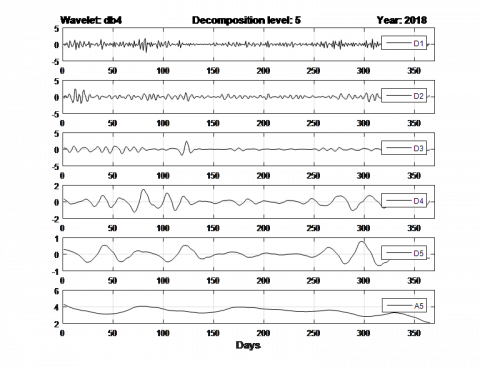Figure 4. 5-level decomposition of daily wind speed data of Annaba for year 2018

## 5.png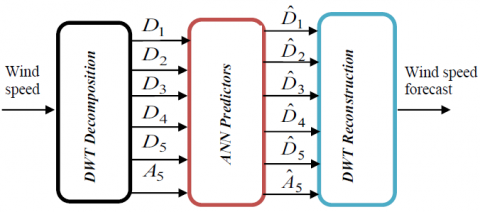Figure 5. Architecture of wavelet-based ANN model

For the employed hybrid forecasting scheme, employing the DWT and a NN as depicted in Figure 5, the following points are considered:

A sliding window of size w=5, representing the number of previous daily average wind speeds (days: d, d-1, d-2, d-3, d-4 and d-5) used to predict the next (sixth day) day (d+1) average wind speed.

Each approximation and detail coefficients, and A5, has its own neural network as shown in Figure 6. All the six networks consist of five inputs, one output and two hidden layers having 10 neurons each.

From the twelve years 2007-2018 of collected daily wind speed, a dataset of size 4375 samples is obtained, from which 4015 (years 2007-2017) and 360 (year 2018) data samples are respectively used for training and testing the network.

## 6.png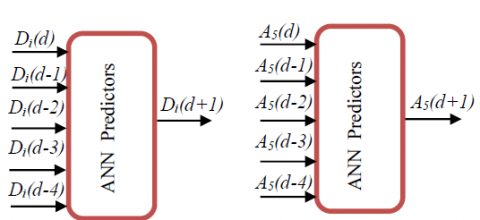Figure 6. ANN structure for estimating Di(d+1) with i=1,2,3, 4, 5and A5(d+1)

This paper proposes a hybrid model based on the combination of the best (discrete) wavelet techniques (DWT) and neural network (WNN) to predict the wind speed of the next day using a database of wind speed spotted on 13 years.

To justify the accuracy and performance of any predictive model, several error measures are used. In this paper, the forecasting performance of the employed scheme is measured using the root mean square error (RMSE) and the mean absolute percentage error (MAPE) described by Eqns. (6) and (7), respectively :

$R M S E=\sqrt{\frac{\sum_{i=1}^{N}\left(x_{i}-\hat{x}_{i}\right)^{2}}{N}}$              (6)

$M A P E=\frac{100 \%}{N} \sum_{i=1}^{N}\left|\frac{x_{i}-\hat{x}_{i}}{x_{i}}\right|$                (7)

where, $x_{i}$ and $\hat{x}_{i}$ represent the measured and estimated wind speed data respectively.

5. Simulation Results and Analysis

The employed hybrid forecasting structure includes a DWT transformation with a neural network for wind speed data prediction. In order to evaluate the effectiveness of the considered scheme for finding out the best wavelet, the following steps are executed:

(1) The daily wind speed data series, for all the years 2007-2018, are transformed using 5-level decomposition DWT to obtain the approximation coefficient A5 and the five detail coefficients {D1, D2,  D3,  D4 , D5}.

(2) Once the six neural networks are properly trained, and using the sliding window w=5, estimates of the approximation coefficient $\widehat{\mathrm{A}}_{5}$ and the five detail coefficient $\left\{\hat{D}_{1}, \hat{D}_{2}, \hat{D}_{3}, \hat{D}_{4}, \hat{D}_{5}\right\}$ are computed.

(3) The wind speed data sequence for the year 2018 is estimated:

$\hat{\mathrm{x}}=\widehat{\mathrm{A}}_{5}+\widehat{\mathrm{D}}_{1}+\widehat{\mathrm{D}}_{2}+\widehat{\mathrm{D}}_{3}+\widehat{\mathrm{D}}_{4}+\widehat{\mathrm{D}}_{5}$     (8)

where, $\hat{x}=\left(\hat{x}_{1}, \hat{x}_{2}, \hat{x}_{3}, \ldots \hat{x}_{N}\right)$ is the estimated wind speed data series.

(4) To select the best wavelet in terms of prediction accuracy, the two adopted error criteria are computed.

(5) Steps 1-4 are executed for the three regions using all the considered wavelets.

Table 1. The RMSE and MAPE values for the three regions using a 5-level DWT decomposition

 ANNABA SIDI BOUZID TETOUANE RMSE MAPE RMSE MAPE RMSE MAPE db1 0.6578 0.1274 0.5147 0.1440 0.9747 0.1477 db2 0.2299 0.0525 0.1943 0.0668 0.3813 0.0614 db3 0.1994 0.0466 0.1753 0.0640 0.3484 0.0562 db4 0.1612 0.0371 0.1265 0.0471 0.2576 0.0451 db5 0.2330 0.0570 0.1871 0.0677 0.3328 0.0625 db6 0.2471 0.0593 0.2251 0.0861 0.4101 0.0756 db7 0.1891 0.0444 0.1946 0.0705 0.3616 0.0615 db8 0.2499 0.0593 0.2040 0.0715 0.3586 0.0642 db9 0.2281 0.0542 0.2005 0.0752 0.4036 0.0688 db10 0.2054 0.0507 0.1717 0.0673 0.3287 0.0595 sym1 0.6562 0.1312 0.5139 0.1427 0.9663 0.1457 sym2 0.2361 0.0536 0.1841 0.0631 0.3315 0.0538 sym3 0.1894 0.0440 0.1573 0.0546 0.3509 0.0611 sym4 0.1763 0.0424 0.1494 0.0535 0.2859 0.0481 sym5 0.1759 0.0419 0.1545 0.0537 0.2979 0.0494 sym6 0.2018 0.0500 0.1743 0.0665 0.3472 0.0572 sym7 0.1979 0.0467 0.1504 0.0568 0.3573 0.0574 sym8 0.1948 0.0458 0.1875 0.0653 0.3492 0.0603 sym9 0.2055 0.0462 0.1778 0.0644 0.3557 0.0595 sym10 0.1988 0.0479 0.1724 0.0603 0.3468 0.0588 coif1 0.1797 0.0409 0.1396 0.0481 0.3362 0.0564 coif2 0.2324 0.0591 0.2216 0.0783 0.3723 0.0649 coif3 0.1819 0.0441 0.1569 0.0555 0.3113 0.0535 coif4 0.1520 0.0356 0.1364 0.0475 0.3013 0.0493 coif5 0.1454 0.0339 0.1317 0.0450 0.2804 0.0488

## 7.pngFigure 7. Measured and estimated wind speed using the db4 wavelet with 5-level decomposition for Annaba region

## 8.png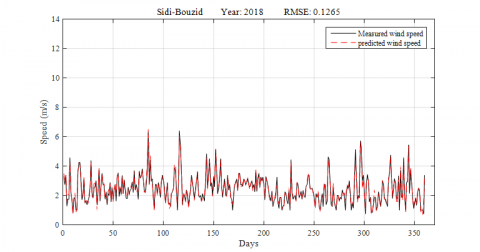Figure 8. Measured and estimated wind speed using the db4 wavelet with 5-level decomposition for Sidi Bouzid region

## 9.png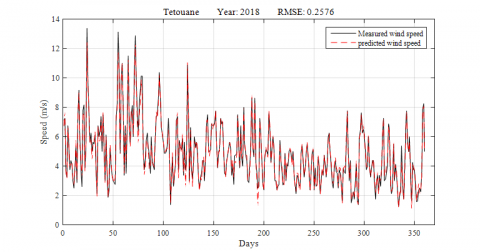Figure 9. Measured and estimated wind speed using the db4 wavelet with 5-level decomposition for Tetouane region

First of all, by conducting a thorough investigation using all the wavelets and the three regions, it has been found that the fifth decomposition level gives practically the best results in terms of wind speed prediction accuracy. Note that, for each simulation execution using a specific wavelet, and in order to avoid the effect of random initialization, the neural network training process is repeated 100 times and the best results are considered. Table 1 reports the RMSE and MAPE error values for the three considered regions using the Daubechies, symlet and coiflet orthogonal wavelet families. From the obtained simulation results, the coif5 shows the best performance for the region of Annaba. Yet, the db4 outperforms, globally, all the tested wavelets for the three regions in terms of forecasting accuracy. To further show the efficiency of the employed forecasting model, the predicted and the measured wind speed data, using the db4, for the three regions are illustrated in Figures 7-9. It is clear that the predicted speed data obtained through the proposed hybrid DWT-ANN method are in good agreement with the actual speed data for practically the whole year 2018. Also, the suggested scheme is able to predict wind speed with significant accuracy showing the least (RMSE, MAPE) values of (0.1612, 0.0371), (0.1265, 0.0471) and (0.2576, 0.0451) for the regions of Annaba, Sidi Bouzid and Tetouane, respectively.

6. Conclusion

Wind speed forecasting plays a significant role in power generation planning and optimization of wind farms. Thus, many techniques relevant to wind speed prediction, have been proposed, where it has been shown that their forecasting effectiveness depends greatly on the data preprocessing step. In this sense, this works aims at forecasting the average daily wind speed using a predicting scheme based on discrete wavelet transform (DWT) associated with Artificial Neural Networks (ANN). The DWT, employed to preprocess the signal, decompose the time series into a set of sub-series to boost further the prediction accuracy. Thus, many wavelet families, including Daubechies, Symlet, and Coiflet discrete wavelets have been tested. To assess the performance of the proposed scheme, wind speed data collected from three regions, namely Annaba, Sidi Bouzid and Tetouane from 2007 to 2018, have been used. After a thorough analysis, the obtained results show that, when using ANN for prediction, the db4 wavelet with a 5-level decomposition is the most appropriate wavelet for wind speed forecasting in the three considered regions. Also the proposed scheme exhibits high precision and accuracy for one day ahead wind speed forecasting in terms of RMSE and MAPE index errors.

Acknowledgment

This work is supported by the Directorate General of Scientific Research and Technological Development (DGRSDT), Algeria.

References

 Liu, H., Duan, Z., Li, Y., Lu, H. (2018). A novel ensemble model of different mother wavelets for wind speed multistep forecasting. Applied Energy, 228: 783-1800. https://doi.org/10.1016/j.apenergy.2018.07.050

 Qian, Z., Pei, Y., Zareipour, H., Chen, N. (2019). A review and discussion of decomposition-based hybrid models for wind energy forecasting applications. Applied Energy, 235: 939-953. https://doi.org/10.1016/j.apenergy.2018.10.080

 Wang, X.C., Guo, P., Huang, X.B. (2011). A review of wind power forecasting models. Energy Procedia, 12: 770-778. https://doi.org/10.1016/j.egypro.2011.10.103

 Dhiman, H.S., Pritam, A., Dipankar, D. (2019). Wavelet transform and variants of SVR with application in wind forecasting. Springer Nature. Innovations in Infrastructure, Advances in Intelligent Systems and Computing, 757: 501-511. https://doi.org/10.1007/978-981-13-1966-2_45

 Xie, H., Ding, M., Chen, L., An, J., Chen, Z., Wu, M. (2017). Short-term wind power prediction by using empirical mode decomposition based GA-SYR. Proceedings of the 36th Chinese Control Conference, Dalian, China, pp. 1934-1978. https://doi.org/10.23919/ChiCC.2017.8028818

 Mi, X.W., Liu, H., Li, Y.F. (2017). Wind speed forecasting method using wavelet, extreme learning machine and outlier correction algorithm. Energy Conversion and Management, 151: 709-722. http://dx.doi.org/10.1016/j.enconman.2017.09.034

 Wang, H.Z., Lei, Z.X., Zhang, X., Zhou, B., Peng, J.C. (2019). A review of deep learning for renewable energy forecasting. Energy Conversion and Management 198: 111799. https://doi.org/10.1016/j.enconman.2019.111799

 Aasim, Singh, S.N., Abheejeet, M. (2019). Repeated wavelet transform based ARIMA Model for very short-term wind speed forecasting. Renewable Energy, 136: 1-28. https://doi.org/10.1016/j.renene.2019.01.031

 Deb, C., Zhang, F., Yang, J., Lee, S.E., Shah, K.W. (2017). A review on time series forecasting techniques for building energy consumption. Renewable and Sustainable Energy Reviews, 74: 902-924. http://dx.doi.org/10.1016/j.rser.2017.02.085

 Cavallo, J., Marinescu, A., Dusparic, I., Clarke, S. (2015). Evaluation of forecasting methods for very small-scale networks. Springer International Publishing Switzerland, 9518: 56-75. https://doi.org/ 10.1007/978-3-319-27430-0_5

 Tran, V.G., Grieu, S., Polit, M., Tuan, T.Q., Linh, T.H. (2009). Forecasting of wind speed using wavelets analysis and cascade-correlation neural networks. European Wind Energy Conference and Exhibition (EWEC), Marseille, France.

 Potter, C.W., Negnevitsky, M. (2006). Very short-term wind forecasting for Tasmanian power generation. IEEE Transactions on Power Systems, 21(2): 965-972. https://doi.org/10.1109/TPWRS.2006.873421

 Ferreira, M., Santos, A., Lucio, P. (2019). Short-term forecast of wind speed through mathematical models. Energy Reports, 5: 1172-1184. https://doi.org/10.1016/j.egyr.2019.05.007

 Kaur, T., Kumar, S., Segal, R. (2016). Application of artificial neural network for short term wind speed forecasting. International Conference on Power and Energy Systems, Bangalore, India. https://doi.org/10.1109/PESTSE.2016.7516458

 Monfet, D., Arkhipova, E., Choinière, D. (2013).  Evaluation of a case-based reasoning energy prediction tool for commercial buildings. Proceedings of the 13th International Conference for Enhanced Building Operations, Montreal, Quebec. http://hdl.handle.net /1969.1/151419

 Meng, A., Ge, J., Yin, H., Chen, S. (2016). Wind speed forecasting based on wavelet packet decomposition and artificial neural networks trained by crisscross optimization algorithm. Energy Conversion and Management, 114: 75-88. http://doi.org/10.1016/j.enconman.2016.02.013

 Liu, D., Niu, D.X., Wang, H., Fan, L.L. (2014). Short-term wind speed forecasting using wavelet transform and support vector machines optimized by genetic algorith, Renewable Energy, 62: 592-597. http://doi.org/10.1016/j.renene.2013.08.011

 Mandal, P., Zareipour, H., Rosehart, W.D. (2014). Forecasting aggregated wind power production of multiple wind farms using hybrid wavelet PSO-NNs. International Journal of Energy Research, 38: 1654-1666. https://doi.org/10.1002/er.3171

 Prema, V., Rao, K.U., Jnaneswar., B.S., Badarish., C.A., Ashok., P.S., Agarwal, S. (2016). Application of hybrid neuro-wavelet models for effective prediction of wind speed. Intelligent Systems Technologies and Applications, Springer International Publishing Switzerland, 384: 345-354. https://doi.org/ 10.1007/978-3-319-23036-8_30

 Rocha Reis, A.J., Alves da Silva, A.P. (2005). Feature extraction via multiresolution analysis for, short-term load forecasting. IEEE Transactions on Power Systems, 20(1): 189-198. https://dx.doi.org/10.1109/TPWRS.2004.840380

 Amjady, N., Keynia, F. (2009). Short-term load forecasting of power systems by combination of wavelet transform and neuro-evolutionary algorithm. Energy, 34(1): 46-57. https://doi.org/10.1016/j.energy.2008.09.020

 Amjady, N., Keynia, F. (2008). Day ahead price forecasting of electricity markets by a mixed data model and hybrid forecast method. International Journal of Electrical Power & Energy Systems, 30(9): 533-546. https://doi.org/10.1016/j.ijepes.2008.06.001

 Catalao, J.P.S., Pousinho, H.M.I., Mendes, V.M.F. (2011). Hybrid wavelet-PSO-ANFIS approach for short-term electricity prices forecasting. IEEE Transactions on Power Systems, 26(1): 137-144. https://doi.org/10.1109/TPWRS.2010.2049385

 Catalão, J.P.S., Pousinho, H.M.I., Mendes, V.M.F. (2011). Short-term wind power forecasting in Portugal by neural networks and wavelet transform. Renewable Energy, 36: 1245-1251. https://doi.org/10.1016/j.renene.2010.09.016

 Ma, Y.J., Zhai, M.Y. (2019). A dual-step integrated machine learning model for 24h-ahead wind energy generation prediction based on actual measurement data and environmental factors. Applied Sciences, 9: 2125. https://doi.org/10.3390/app9102125

 Addison, S.P. (2017). The Illustrated Wavelet Transform Handbook: Introductory Theory and Applications in Science. Engineering, Medicine and Finance, by Taylor & Francis Group, CRC Press. ISBN: 9781315372556. https://doi.org/10.1201/9781315372556

 Yan, R.Q., Gao, R.X., Chen, X.F. (2014). Wavelets for fault diagnosis of rotary machines: A review with applications, Signal Processing, 96(part A): 1–15. http://doi.org/10.1016/j.sigpro.2013.04.015

 https://rp5.ru/Weather_archive_in_Sidi_Bou_Zid, accessed on 9 October 2019.